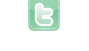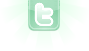## Reflection law calculate new direction (Tank Game)

1
2
3
In my Tank Game your bullets bounce once if they hit a wall (just like a pool ball). My problem is to figure out the following two things:
```
if(getOneIntersectingObject(Wall.class) != null && bounceCounter < 2) { //if touches wall and bounced less than 2 times
Wall wall = (Wall) getOneIntersectingObject(Wall.class); //save wall
if(Math.abs(wall.getX()-getX()) < Math.abs(wall.getY()-getY())) { //top bottom side
//setNewRotation!
move(5*QVAL); //get away from wall for next frame
} else if(Math.abs(wall.getX()-getX()) < Math.abs(wall.getY()-getY())) { //left right side
//setNewRotation!
move(5*QVAL); //get away from wall for next frame
}
bounceCounter++;
return true;
}

```
Here i check whether i hit a wall from the left/right or top/bottom (is this needed? is this done the right way? My world is 1200 x 800 large). My second problem is the actual formula. I want to setRotation(someValue) dependent on getRotation(). So it should look like something like this: setRotation(getRotation()-bla+blabla*something%idontknow). Is checking if i hit left, right, top, bottom of the border walls necessary? If yes, do i check the right way with th world being rectangular (1200x800)? What is the formula for the new rotation dependent on the current/old rotation? Thank you!
You will need to know whether your tank is hitting a horizontal (top/bottom) or vertical (left/right) wall because the formulas for the changes in rotation are different. In general:
```// for top/bottom
setRotation(-getRotation());

// for left/right
setRotation(180-getRotation());```
Your given code could not possibly be right as between lines 3 and 9 you have the exact same code on both sides of else. You will probably have to undo the initial move (when a wall is detected) and perform horizontal and vertical movement separately to determine which wall(s) were encountered. This would involve breaking the move vector up into its side length components and "bouncing" off the amount of distance the wall was penetrated.
Alright i think i understand. When intersecting with the wall i need to get the length of the vector from tank to the intersectedpoint (how to i get the coords for the intersection???). Then move the length of that vector, setRotation to the new value and then again move the length of the vector. I hope i did get you right here? Or is your solution different? Because in the actor API there seems to be no such method as getOnesIntersectionCoords(some.class). And the getX(), getY() of the wall are the center of the 40x40 image instead of the wanted edge that the shell is intersecting with. Thanks!
I am not sure that you do understand. The vector referred to is the combination of the actor's rotation and how fast it moves. It is apparently moving at a speed of exactly 500 units (a unit being 1/100 of a pixel dimension -- 1/QVAL). This "angle/length" vector can be seen as an hypotenuse of a triangle on a standard coordinate grid with sides, one equal to the amount of horizontal movement (dx) and the other the amount of vertical movement (dy):
```int dx = (int)(Math.cos(Math.PI*getQR()/QVAL/180)*5*QVAL);
int dy = (int)(Math.sin(Math.PI*getQR()/QVAL/180)*5*QVAL);```
After initial move and finding that the actor intersects a wall, you would immediately move back the full amount and then individually move horizontally and vertically the calculated amounts, checking for intersection after each. The distance to bounce back would be double the distance between the actor's current location and location of the edge of the wall. The signs (+/-) of dx and dy will determine which side of a wall was hit and what is needed to calculate the new position.
I understand gut in greenfoot there is nothing like getIntersecting point. Also I can't get the intersected side of the 40 × 40 pixel wall by comparing the x and y offsets. So how can I tell which side of a 40×40 box in the center of the worId, I've hit. Thank you for your kind help.
SushiLlama wrote...
I understand gut in greenfoot there is nothing like getIntersecting point. Also I can't get the intersected side of the 40 × 40 pixel wall by comparing the x and y offsets. So how can I tell which side of a 40×40 box in the center of the worId, I've hit. Thank you for your kind help.
That is one of the points of breaking the move vector up into its constituent pieces. So that if you move in a direction along an axis and come into contact with a wall, you know which side you have hit (e.g. if moving horizontally and dx is negative, you moved left into the right side of a wall).
Omg yes, thanks you genius. I am going to test it right after my lesson. Thank you so much for helping everybody here!
danpost wrote...
The distance to bounce back would be double the distance between the actor's current location and location of the edge of the wall.
I understand what you mean but how to you get the location of the edge of the wall? There is no getIntersectingPoint() method for the Actor. You could use the half of the image width of the shell but this would be not very accurate when thinking about small angle bounces :( Any suggestion? Here is my code: (I think it's right (is my QVAL usage correct???) but i am still missing methods to get the intersection location)
```    public boolean checkBounce() {
if (getOneIntersectingObject(Wall.class) != null && bounceCounter < 2) { //if touches wall and bounced less than 2 times
move(-speed * QVAL); //undo move

int dx = (int) (Math.cos(Math.PI * getQR() / QVAL / 180) * speed * QVAL);
int dy = (int) (Math.sin(Math.PI * getQR() / QVAL / 180) * speed * QVAL);
int intersectionX = getIntersectionX();
int intersectionY = getIntersectionY();
int newMovingVectorLength;
int newRotation = -1;

if (checkVerticalBounce(dx)) {
newRotation = -getRotation();
} else if (checkHorizontalBounce(dy)) { //Unnecessary. If not checkVerticalBounce then checkHorizontalBounce must be true, but yeah...
newRotation = 180-getRotation();
}

newMovingVectorLength = (int) Math.sqrt(Math.pow(intersectionX, 2) + Math.pow(intersectionY, 2));
move(newMovingVectorLength*QVAL);
setQRotation(newRotation*QVAL);
move(newMovingVectorLength*QVAL);
bounceCounter++;
return true;
}
return false;
}

//What am i supposed to do here :(
public int getIntersectionX() {
return -1;
}
public int getIntersectionY() {
return -1;
}

public boolean checkVerticalBounce(int dx) {
move(dx * QVAL);
if (getOneIntersectingObject(Wall.class) != null) {
//You've hit the top or bottom of the wall
setRotation((180 - getRotation()) % 360);
move(-dx * QVAL);
return true;
} else {
move(-dx * QVAL);
return false;
}
}

public boolean checkHorizontalBounce(int dy) {
move(dy * QVAL);
if (getOneIntersectingObject(Wall.class) != null) {
//You've hit the left or right side of the wall
setRotation((180 - getRotation()) % 360);
move(-dy * QVAL);
return true;
} else {
move(-dy * QVAL);
return false;
}
}
```
SushiLlama wrote...
is my QVAL usage correct?
Certainly not, with respect to dx and dy. These are already in units of 1/QVAL and should not be multiplied again. I was thinking along these lines:
```move(5*QVAL); // initial moving
if (isTouching(Wall.class)
{
move(-5*QVAL); // moving back
// horizontal moving
int dx = (int) (Math.cos(Math.PI * getQR() / QVAL / 180) * speed * QVAL);
setQLocation(getQX()+dx, getQY());
Actor wall = getOneIntersectingObject(Wall.class);
if (wall != null)
{
int dir = (int) Math.signum(dx);
int dist = (wall.getImage().getWidth()+this.getImage().getWidth())*QVAL/2+99-Math.abs(wall.getX()*QVAL-getQX());
setQLocation(getQX()-dir*dist, getQY());
}
// vertical moving
int dy = (int) (Math.sin(Math.PI * getQR() / QVAL / 180) * speed * QVAL);
setQLocation(getQX(), getQY()+dy);
Actor wall = getOneIntersectingObject(Wall.class);
if (wall != null)
{
int dir = (int) Math.signum(dy);
int dist = (wall.getImage().getHeight()+this.getImage().getHeight())*QVAL/2+99-Math.abs(wall.getY()*QVAL-getQY());
setQLocation(getQX(), getQY()-dir*dist,);
}
}```
This was not tested. There may be typos or bugs. However, you should get the general idea from it.
danpost wrote...
SushiLlama wrote...
is my QVAL usage correct?
Certainly not, with respect to dx and dy. These are already in units of 1/QVAL and should not be multiplied again. I was thinking along these lines:
```move(5*QVAL); // initial moving
if (isTouching(Wall.class)
{
move(-5*QVAL); // moving back
// horizontal moving
int dx = (int) (Math.cos(Math.PI * getQR() / QVAL / 180) * speed * QVAL);
setQLocation(getQX()+dx, getQY());
Actor wall = getOneIntersectingObject(Wall.class);
if (wall != null)
{
int dir = (int) Math.signum(dx);
int dist = (wall.getImage().getWidth()+this.getImage().getWidth())*QVAL/2+99-Math.abs(wall.getX()*QVAL-getQX());
setQLocation(getQX()-dir*dist, getQY());
}
// vertical moving
int dy = (int) (Math.sin(Math.PI * getQR() / QVAL / 180) * speed * QVAL);
setQLocation(getQX(), getQY()+dy);
Actor wall = getOneIntersectingObject(Wall.class);
if (wall != null)
{
int dir = (int) Math.signum(dy);
int dist = (wall.getImage().getHeight()+this.getImage().getHeight())*QVAL/2+99-Math.abs(wall.getY()*QVAL-getQY());
setQLocation(getQX(), getQY()-dir*dist,);
}
}```
This was not tested. There may be typos or bugs. However, you should get the general idea from it.
I adjusted the code as follows to fit my needs:
```public boolean checkBounce() {
if (getOneIntersectingObject(Wall.class) != null && bounceCounter < 2) {
move(-speed * QVAL); // moving back
// horizontal moving
int dx = (int) (Math.cos(Math.PI * getQR() / QVAL / 180) * speed * QVAL);
setQLocation(getQX() + dx, getQY());
// horizontal check
Actor wall = getOneIntersectingObject(Wall.class);
if (wall != null) {
int dir = (int) Math.signum(dx);
int dist = (wall.getImage().getWidth() + this.getImage().getWidth()) * QVAL / 2 + 99 - Math.abs(wall.getX() * QVAL - getQX());
setQLocation(getQX() - dir * dist, getQY());
}
// vertical moving
int dy = (int) (Math.sin(Math.PI * getQR() / QVAL / 180) * speed * QVAL);
setQLocation(getQX(), getQY() + dy);
//horizontal check
wall = getOneIntersectingObject(Wall.class);
if (wall != null) {
int dir = (int) Math.signum(dy);
int dist = (wall.getImage().getHeight() + this.getImage().getHeight()) * QVAL / 2 + 99 - Math.abs(wall.getY() * QVAL - getQY());
setQLocation(getQX(), getQY() - dir * dist);
}
bounceCounter++;
return true;
}
return false;
}
```
Sadly every shell i shoot, now gets just sucked into the wall when intersecting it. So sadly there is something not working as planned i think.
Ah, yes. I, for one thing, forgot to add the changes in rotation into the code.
danpost wrote...
Ah, yes. I, for one thing, forgot to add the changes in rotation into the code.
Could you fix that? Would be great! Thanks!!! Otherwise I'll try my best!
I just dont got it right:( could you help please?
SushiLlama wrote...
I just dont got it right:( could you help please?
I started testing on the codes. Having a bit of a problem with vertical bouncing and not sure why yet. Still working on it. Currently, I have this:
```move(speed*QVAL);
if (isTouching(Wall.class))
{
move(-speed*QVAL);
int dx = (int) (Math.cos(Math.PI*getQR()/QVAL/180)*speed*QVAL);
setQLocation(getQX()+dx, getQY());
Actor wall = getOneIntersectingObject(Wall.class);
if (wall != null)
{
int dir = (int) Math.signum(dx);
int dist = (wall.getImage().getWidth()+getImage().getWidth())*QVAL/2+(QVAL-1)-Math.abs(wall.getX()*QVAL-getQX());
setQLocation(getQX()-dir*dist, getQY());
setQRotation(180*QVAL-getQR());
}
int dy = (int) (Math.sin(Math.PI*getQR()/QVAL/180)*speed*QVAL);
setQLocation(getQX(), getQY()+dy);
wall = getOneIntersectingObject(Wall.class);
if (wall != null)
{
int dir = (int) Math.signum(dy);
int dist = (wall.getImage().getHeight()+getImage().getHeight())*QVAL/2+(QVAL-1)-Math.abs(wall.getY()*QVAL-getQY());
setQLocation(getQX(), getQY()-dir*dist);
setQRotation(-getQR());
}
}```
I have basically the same codes for a vertical bounce as for an horizontal bounce. Only major difference is the formula for change in rotation -- and I know that it is correct. Not sure why the actor is not backed off the wall properly when moving vertically. Line 22 should take care of that; but, even then, it does not appear to back off at all.
Oh, duh. I know what is going on. I did not take into account the actual rotation of the act. The width and height along the horizontal and vertical are not the normal width and height when the actor is rotated. It might be easiest to assume maximum length for all directions. This would simply end up to be this:
```move(speed*QVAL);
if (isTouching(Wall.class))
{
move(-speed*QVAL);
int dx = (int) (Math.cos(Math.PI*getQR()/QVAL/180)*speed*QVAL);
move(dx, 0);
Actor wall = getOneIntersectingObject(Wall.class);
if (wall != null)
{
int dir = (int) Math.signum(dx);
int dist = (wall.getImage().getWidth()+getImage().getWidth())*QVAL/2+QVAL-Math.abs(wall.getX()*QVAL-getQX());
setQLocation(getQX()-dir*dist, getQY());
setQRotation(180*QVAL-getQR());
}
int dy = (int) (Math.sin(Math.PI*getQR()/QVAL/180)*speed*QVAL);
move(dy, 90*QVAL);
wall = getOneIntersectingObject(Wall.class);
if (wall != null)
{
int dir = (int) Math.signum(dy);
int dist = (wall.getImage().getHeight()+getImage().getWidth())*QVAL/2+QVAL-Math.abs(wall.getY()*QVAL-getQY());
setQLocation(getQX(), getQY()-dir*dist);
setQRotation(-getQR());
}
}```
I just used the the actor's width n the calculation of dist in the vertical bounce code.
There are more replies on the next page.
1
2
3